# NCERT Solutions for Class 7 Maths Chapter 9 Rational Numbers Ex 9.2

NCERT Solutions for Class 7 Maths Chapter 9 Rational Numbers Ex 9.2

### NCERT Solutions for Class 7 Maths Chapter 9 Rational Numbers Ex 9.2

NCERT Solutions for Class 7 Maths Chapter 9 Rational Numbers Exercise 9.2
Ex 9.2 Class 7 Maths Question 1.
Find the sum: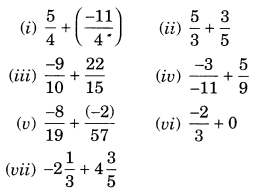Solution.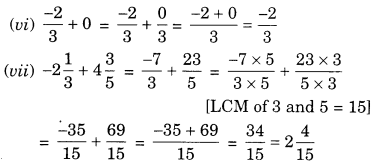Ex 9.2 Class 7 Maths Question 2.
Find: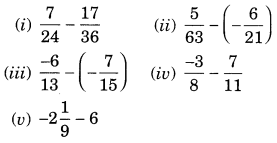Solution: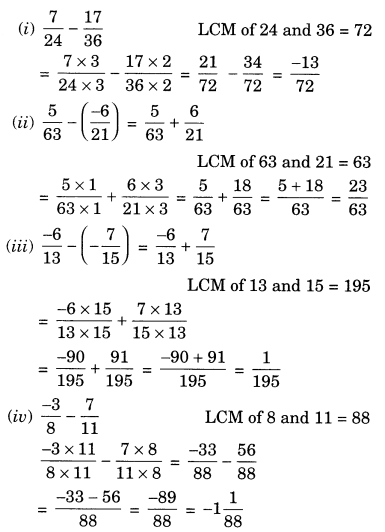Ex 9.2 Class 7 Maths Question 3.
Find the product:Solution:Ex 9.2 Class 7 Maths Question 4.
Find the value of:Solution: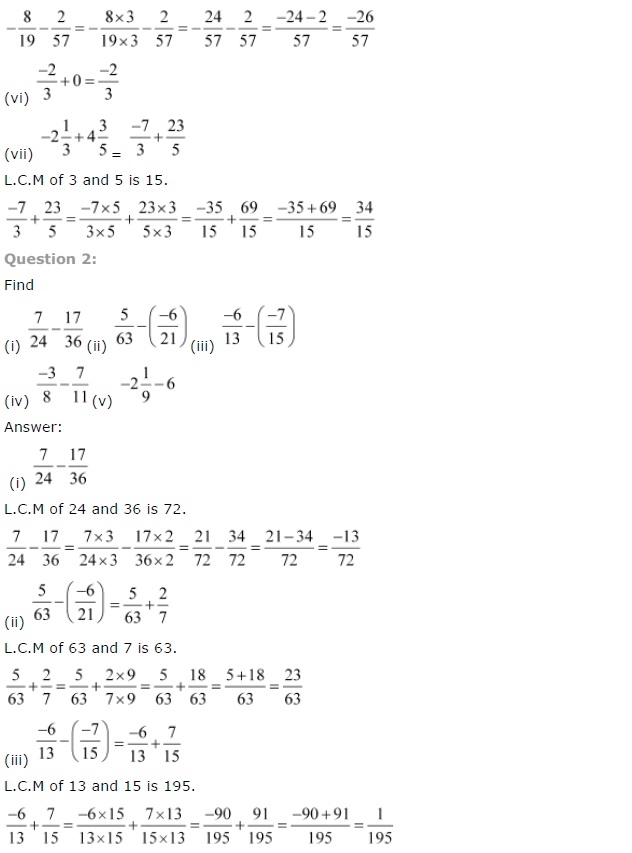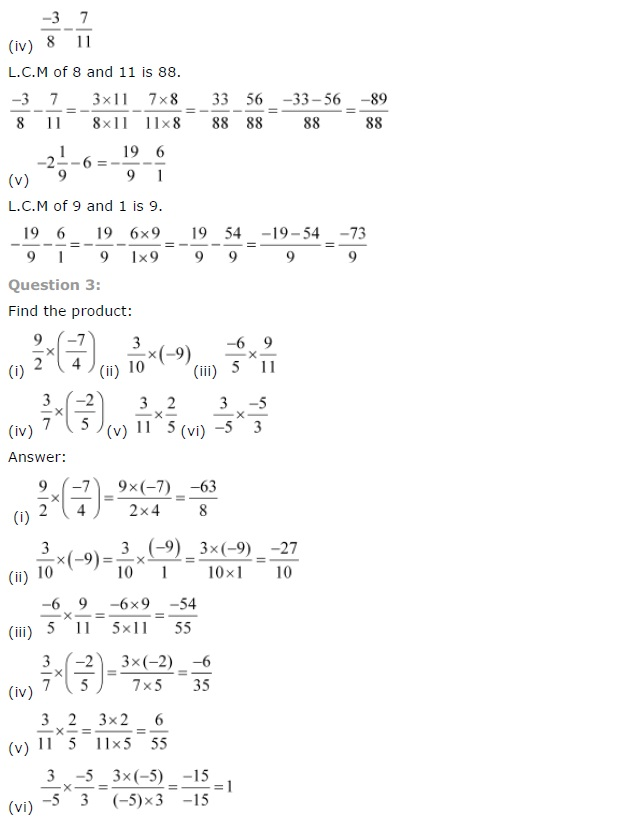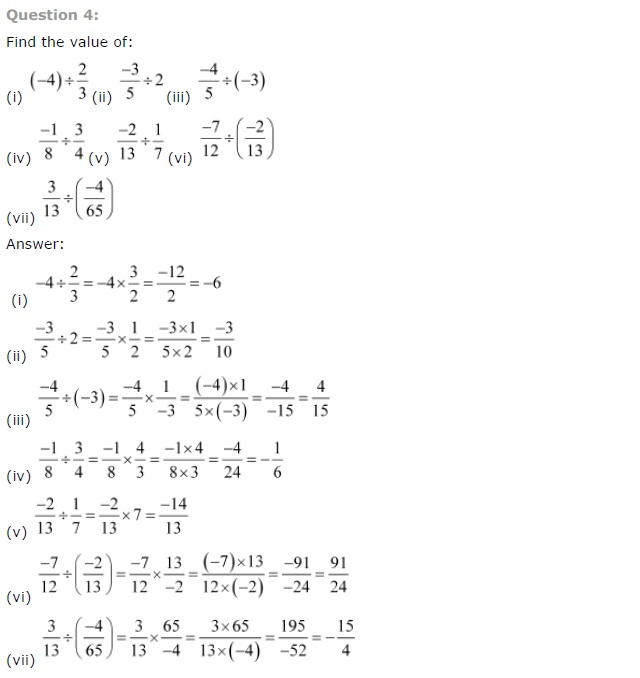## SabDekho

The Complete Educational Website